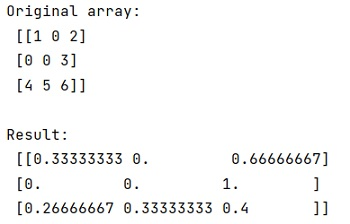# Normalize a SciPy Sparse Matrix

In this tutorial, we will learn about an efficient way to normalize a SciPy sparse matrix with the help of example. By Pranit Sharma Last updated : April 19, 2023

## What Does Normalize a SciPy Sparse Matrix Mean?

Normalizing a matrix means scaling a value of the matrix in such a way that the range of rows and columns lies between 1 and 0.

Here, we have a SciPy sparse matrix, and we need to normalize this matrix.

## How to Normalize a SciPy Sparse Matrix?

To normalize a SciPy sparse matrix, you can simply use sklearn.preprocessing.normalize() method which is used to normalize vectors or matrices. It takes a parameters norm which is the norm to use to normalize each non-zero sample.

### Syntax

Use the following syntax to normalize a SciPy sparse matrix:

```sklearn.preprocessing.normalize(X, norm='l2', *, axis=1, copy=True, return_norm=False)
```

## Python Program to Normalize a SciPy Sparse Matrix

```# Import numpy
import numpy as np

# Import scipy sparse
import scipy.sparse as sp

# Import scikit-learn preprocessing
from sklearn.preprocessing import normalize

# Creating a sparse matrix
row = np.array([0, 0, 1, 2, 2, 2])
col = np.array([0, 2, 2, 0, 1, 2])
data = np.array([1, 2, 3, 4, 5, 6])
arr = sp.csr_matrix((data, (row, col)), shape=(3, 3)).toarray()

# Display original array
print("Original array:\n", arr, "\n")

# Normalizing matrix
res = normalize(arr, norm="l1", axis=1)

# Display result
print("Result:\n", res)
```

### Output